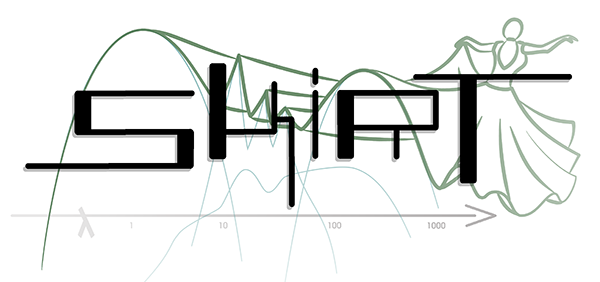SKIRT 9 advanced radiative transfer in dusty systems
VoigtProfile Namespace Reference

## Functions

double sample (double a, double x, Random *random)

double value (double a, double x)

## Detailed Description

This namespace offers functions related to the Voigt profile, which is a probability distribution given by a convolution of a Cauchy-Lorentz distribution and a Gaussian distribution.

## ◆ sample()

 double VoigtProfile::sample ( double a, double x, Random * random )

This function samples a random value from the probability distribution $$P(u)$$ defined by

$P(u) \propto \frac{\mathrm{e}^{-u^2}}{(u-x)^2+a^2}$

where $$a$$ and $$x$$ are parameters given as arguments and the proportionality factor is determined by normalization. The third argument specifies the random generator to be used by the function.

We use the method described by Zheng et al. 2002 (ApJ, 578, 33-42, appendix), a variation of which is also used by many other authors including Tasitsiomi 2006 (ApJ, 645, 792-813), Laursen et al. 2009 (ApJ, 696, 853-869), Smith et al. 2015 (MNRAS, 449, 4336–4362) and Michel-Dansac et al. 2020 (A&A). The method is based on the rejection technique for sampling from a probability distribution. One could use the comparison function $$g(u)\propto \left[ (u-x)^2 + a^2 \right]^{-1}$$ which can be integrated and inverted analytically so that it can be sampled using $$u= x + a \tan \left[ \frac{\pi}{2} (2\mathcal{X}-1) \right]$$, with $$\mathcal{X}$$ a uniform deviate.

Because of the peculiar shape of $$P(u)$$, however, the comparison function $$g(u)$$ is better defined as

$g(u) \propto \begin{cases} \left[ (u-x)^2 + a^2 \right]^{-1} & u \le u_0 \\ \mathrm{e}^{-u_0^2}\left[ (u-x)^2 + a^2 \right]^{-1} & u > u_0 \end{cases}$

where $$u_0$$ is determined following Michel-Dansac et al. 2020 (A&A) by

\begin{align*} u_0 = &\; 2.648963+2.014446 \zeta+0.351479\zeta^2 \\ &+ x (-4.058673-3.675859\zeta-0.640003\zeta^2 \\ &+ x (3.017395+2.117133\zeta+0.370294\zeta^2 \\ &+ x (-0.869789-0.565886\zeta-0.096312\zeta^2 \\ &+ x (0.110987+0.070103\zeta+0.011557\zeta^2 \\ &+ x (-0.005200-0.003240\zeta-0.000519\zeta^2))))) \end{align*}

where $$\zeta = \log_{10}(a)$$.

The acceptance fractions are required to be $$\mathrm{e}^{-􏰜u^2}$$ and $$\mathrm{e}^{-􏰜u^2} / \mathrm{e}^{-􏰜u_0^2}$$ in the regions $$u\le 􏰠u_0$$ and $$u>u_0$$, respectively. A first random number uniformly distributed between 0 and 1 determines which region we use by comparing it with p, where

$p = \frac{\int_{-\infty}^{u_0} g(u)\,\mathrm{d}u} {\int_{-\infty}^{+\infty} g(u)\,\mathrm{d}u} = \left( \theta_0 + \frac{\pi}{2} \right) \left[ \left( 1-\mathrm{e}^{-u_0^2} \right) \theta_0 + \left( 1+\mathrm{e}^{-u_0^2} \right) \frac{\pi}{2} \right]^{-1}, \quad \theta_0 = \arctan \frac{u_0 -x}{a} .$

Finally, for larger values of $$x$$, the distribution $$P(u)$$ can successfully be approximated by a Gaussian distribution centered on $$1/x$$. Following Smith et al. 2015 (MNRAS, 449, 4336–4362) and Michel-Dansac et al. 2020 (A&A), we use this approximation for $$x \ge 8$$.

## ◆ value()

 double VoigtProfile::value ( double a, double x )

This function returns (an approximation to) the value of the Voigt function $$H(a,x)$$ defined by

$H(a,x) = \frac{a}{\pi} \int_{-\infty}^\infty \frac{\mathrm{e}^{-y^2}\,\mathrm{d}y}{(y-x)^2+a^2} \approx \begin{cases} \mathrm{e}^{-x^2} & \text{core} \\ \dfrac{a}{\sqrt{\pi}x^2} & \text{wings} \end{cases}$

where $$a$$ is a parameter and the function is normalized so that $$H(a,0) \approx 1$$ for $$a\ll 1$$. For reference, the following table lists $$H(a,0)$$ for a few values of $$a$$.

$$a$$ $$H(a,0)$$
0.03 0.967029
0.01 0.988815
! 0.001 0.998873
! 0.0001 0.999887

We use the approximation provided by Smith et al. 2015 (MNRAS, 449, 4336–4362) in their Appendix A1 and Table A1. According to the authors and as confirmed in the analysis by Michel-Dansac et al. 2020 (A&A), this approximation is accurate to within 1 per cent for all $$x$$ as long as $$a<0.03$$, which for the calculation of the Lyman-alpha cross section corresponds to a gas temperature above the cosmic microwave background temperature, i.e. $$T_\mathrm{gas}>T_\mathrm{CMB}$$. The accuracy improves substantially for lower values of $$a$$, i.e. for higher gas temperatures.